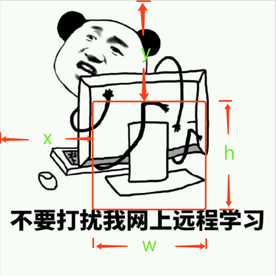# Python图片裁剪实例代码

（距离图片左边界距离x， 距离图片上边界距离y，距离图片左边界距离+裁剪框宽度x+w，距离图片上边界距离+裁剪框高度y+h）``````# -*-coding:utf-8-*-
from PIL import Image
im = Image.open("renren.jpeg")
# 图片的宽度和高度
img_size = im.size
print("图片宽度和高度分别是{}".format(img_size))
'''

'''
# 截取图片中一块宽和高都是250的
x = 100
y = 100
w = 250
h = 250
region = im.crop((x, y, x+w, y+h))
region.save("./crop_test1.jpeg")

# 截取图片中一块宽是250和高都是300的
x = 100
y = 100
w = 250
h = 300
region = im.crop((x, y, x+w, y+h))
region.save("./crop_test2.jpeg")

``````